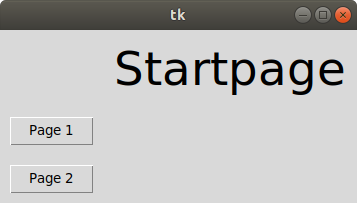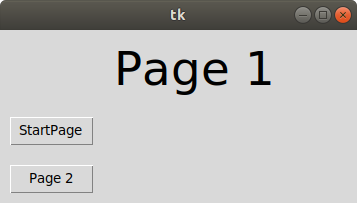# Tkinter Application to Switch Between Different Page Frames

• Difficulty Level : Medium
• Last Updated : 15 Feb, 2021

Prerequisites: Python GUI – tkinter

Sometimes it happens that we need to create an application with several pops up dialog boxes, i.e Page Frames. Here is a step by step process to create multiple Tkinter Page Frames and link them! This can be used as a boilerplate for more complex python GUI applications like creating interfaces for Virtual Laboratories for experiments, classrooms, etc.

Here are the steps:

• Create three different pages. Here we have three different pages, The start page as the home page, page one, and page two.
• Create a container for each page frame.
• We have four classes. First is the tkinterApp class, where we have initialized the three frames and defined a function show_frame which is called every time the user clicks on a button.
• The StartPage is simple with two buttons to go to Page 1 and Page 2.
• Page 1 has two buttons, One for Page 2 and another to return to Start Page.
• Page 2 also has two buttons, one for Page 1 and others to return to StartPage.
• This is a simplistic application of navigating between Tkinter frames.
• This can be used as a boilerplate for more complex applications and several features can be added.

The App starts with the StartPage as the first page, as shown in class tkinterApp. Here in StartApp, there are two buttons. Clicking on a button takes you to the respective Page. You can add images and graphs to these pages and add complex functionality. The pages have two buttons as well. Every time a button is pressed show_frame is called, which displays the respective Page.
Below is the implementation.

## Python3

 `import` `tkinter as tk``from` `tkinter ``import` `ttk`` `  `LARGEFONT ``=``(``"Verdana"``, ``35``)`` ` `class` `tkinterApp(tk.Tk):``    ` `    ``# __init__ function for class tkinterApp``    ``def` `__init__(``self``, ``*``args, ``*``*``kwargs):``        ` `        ``# __init__ function for class Tk``        ``tk.Tk.__init__(``self``, ``*``args, ``*``*``kwargs)``        ` `        ``# creating a container``        ``container ``=` `tk.Frame(``self``) ``        ``container.pack(side ``=` `"top"``, fill ``=` `"both"``, expand ``=` `True``)`` ` `        ``container.grid_rowconfigure(``0``, weight ``=` `1``)``        ``container.grid_columnconfigure(``0``, weight ``=` `1``)`` ` `        ``# initializing frames to an empty array``        ``self``.frames ``=` `{} `` ` `        ``# iterating through a tuple consisting``        ``# of the different page layouts``        ``for` `F ``in` `(StartPage, Page1, Page2):`` ` `            ``frame ``=` `F(container, ``self``)`` ` `            ``# initializing frame of that object from``            ``# startpage, page1, page2 respectively with``            ``# for loop``            ``self``.frames[F] ``=` `frame`` ` `            ``frame.grid(row ``=` `0``, column ``=` `0``, sticky ``=``"nsew"``)`` ` `        ``self``.show_frame(StartPage)`` ` `    ``# to display the current frame passed as``    ``# parameter``    ``def` `show_frame(``self``, cont):``        ``frame ``=` `self``.frames[cont]``        ``frame.tkraise()`` ` `# first window frame startpage`` ` `class` `StartPage(tk.Frame):``    ``def` `__init__(``self``, parent, controller):``        ``tk.Frame.__init__(``self``, parent)``        ` `        ``# label of frame Layout 2``        ``label ``=` `ttk.Label(``self``, text ``=``"Startpage"``, font ``=` `LARGEFONT)``        ` `        ``# putting the grid in its place by using``        ``# grid``        ``label.grid(row ``=` `0``, column ``=` `4``, padx ``=` `10``, pady ``=` `10``)`` ` `        ``button1 ``=` `ttk.Button(``self``, text ``=``"Page 1"``,``        ``command ``=` `lambda` `: controller.show_frame(Page1))``    ` `        ``# putting the button in its place by``        ``# using grid``        ``button1.grid(row ``=` `1``, column ``=` `1``, padx ``=` `10``, pady ``=` `10``)`` ` `        ``## button to show frame 2 with text layout2``        ``button2 ``=` `ttk.Button(``self``, text ``=``"Page 2"``,``        ``command ``=` `lambda` `: controller.show_frame(Page2))``    ` `        ``# putting the button in its place by``        ``# using grid``        ``button2.grid(row ``=` `2``, column ``=` `1``, padx ``=` `10``, pady ``=` `10``)`` ` `         ` ` ` ` ` `# second window frame page1``class` `Page1(tk.Frame):``    ` `    ``def` `__init__(``self``, parent, controller):``        ` `        ``tk.Frame.__init__(``self``, parent)``        ``label ``=` `ttk.Label(``self``, text ``=``"Page 1"``, font ``=` `LARGEFONT)``        ``label.grid(row ``=` `0``, column ``=` `4``, padx ``=` `10``, pady ``=` `10``)`` ` `        ``# button to show frame 2 with text``        ``# layout2``        ``button1 ``=` `ttk.Button(``self``, text ``=``"StartPage"``,``                            ``command ``=` `lambda` `: controller.show_frame(StartPage))``    ` `        ``# putting the button in its place``        ``# by using grid``        ``button1.grid(row ``=` `1``, column ``=` `1``, padx ``=` `10``, pady ``=` `10``)`` ` `        ``# button to show frame 2 with text``        ``# layout2``        ``button2 ``=` `ttk.Button(``self``, text ``=``"Page 2"``,``                            ``command ``=` `lambda` `: controller.show_frame(Page2))``    ` `        ``# putting the button in its place by``        ``# using grid``        ``button2.grid(row ``=` `2``, column ``=` `1``, padx ``=` `10``, pady ``=` `10``)`` ` ` ` ` ` ` ` `# third window frame page2``class` `Page2(tk.Frame):``    ``def` `__init__(``self``, parent, controller):``        ``tk.Frame.__init__(``self``, parent)``        ``label ``=` `ttk.Label(``self``, text ``=``"Page 2"``, font ``=` `LARGEFONT)``        ``label.grid(row ``=` `0``, column ``=` `4``, padx ``=` `10``, pady ``=` `10``)`` ` `        ``# button to show frame 2 with text``        ``# layout2``        ``button1 ``=` `ttk.Button(``self``, text ``=``"Page 1"``,``                            ``command ``=` `lambda` `: controller.show_frame(Page1))``    ` `        ``# putting the button in its place by``        ``# using grid``        ``button1.grid(row ``=` `1``, column ``=` `1``, padx ``=` `10``, pady ``=` `10``)`` ` `        ``# button to show frame 3 with text``        ``# layout3``        ``button2 ``=` `ttk.Button(``self``, text ``=``"Startpage"``,``                            ``command ``=` `lambda` `: controller.show_frame(StartPage))``    ` `        ``# putting the button in its place by``        ``# using grid``        ``button2.grid(row ``=` `2``, column ``=` `1``, padx ``=` `10``, pady ``=` `10``)`` ` ` ` `# Driver Code``app ``=` `tkinterApp()``app.mainloop()`

Output:My Personal Notes arrow_drop_up